# User Forum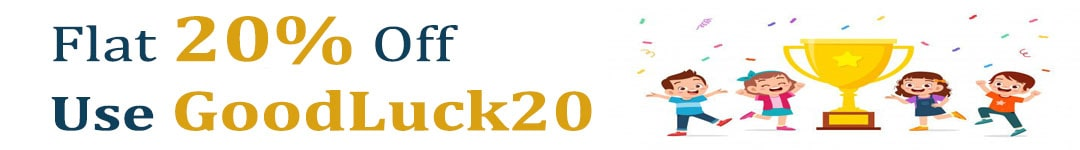Subject :IMO    Class : Class 7

The given question consists of two sets of figure. Figures P, Q, R and S consists of a problem set. There is a definite relationship between figures P and Q. Establish a similar relationship between Figure R and S by selecting a suitable figure from the options that would replace (?) in figure S.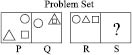(a)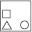(b)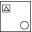(c)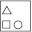(d)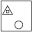explain

Class : Class 7
Ans should be b

## Ans 2:

Class : Class 9
yes triangle should be inside the square so ans is